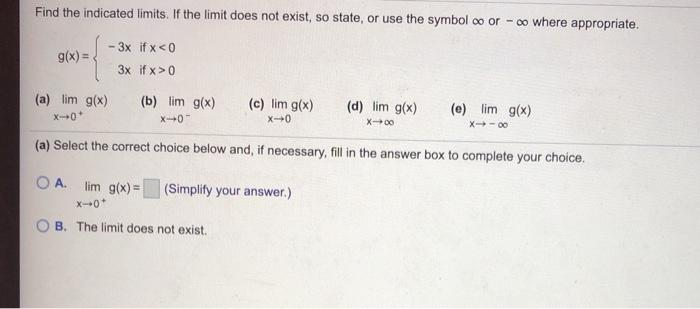### Create an Account

Already have account?

### Forgot Your Password ?

Home / Questions / Find the indicated limits. If the limit does not exist, so state, or use the symbol oo or ...

# Find the indicated limits. If the limit does not exist, so state, or use the symbol oo or -oo where appropriate. g(x) = -3x if x 0 (a) lim g(x) (b) lim g(x) X-01 (c) lim g(x) X+0 (d) lim g(x) (e) lim

Find the indicated limits. If the limit does not exist, so state, or use the symbol oo or -oo where appropriate. g(x) = -3x if x 0 (a) lim g(x) (b) lim g(x) X-01 (c) lim g(x) X+0 (d) lim g(x) (e) lim g(x) X00 (a) Select the correct choice below and, if necessary, fill in the answer box to complete your choice. OA. lim g(x)= (Simplify your answer.) B. The limit does not exist.Apr 19 2021 View more View Less

#### Answer (Solved)Subscribe To Get Solution